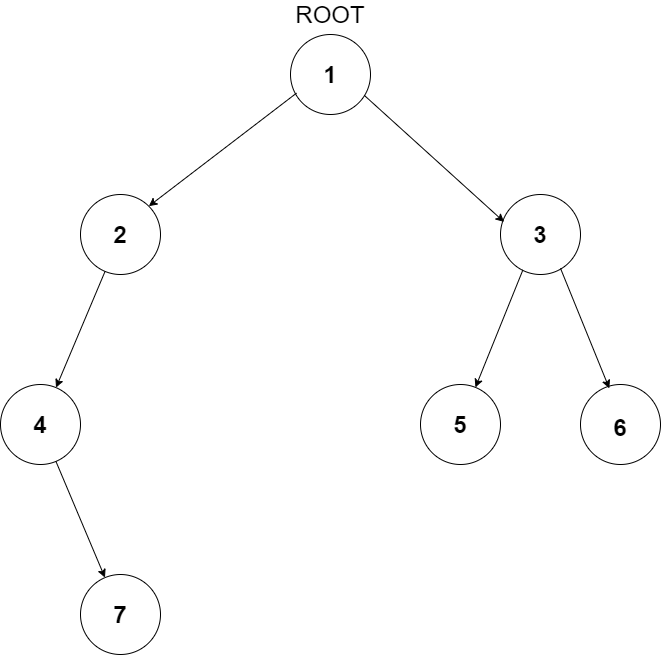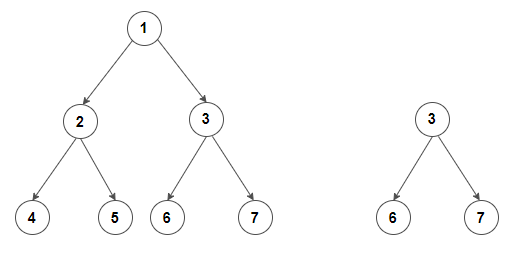6

# Subtree of Another Tree

Difficulty: HARD
Avg. time to solve
10 min
Success Rate
90%

Problem Statement
Suggest Edit

#### A subtree of a tree T is a tree S consisting of a node in T and all of its descendants in T. The subtree corresponding to the root node is the entire tree; the subtree corresponding to any other node is called a proper subtree.

##### Input Format:
``````The first line of input contains an integer ‘T’ representing the number of test cases. Then the test cases follow.

The first line of each test case contains elements of the first tree in the level order form. The line consists of values of nodes separated by a single space. In case a node is null, we take -1 in its place.

The second line of each test case contains elements of the second tree in the level order form. The line consists of values of nodes separated by a single space. In case a node is null, we take -1 in its place.

For example, the input for the tree depicted in the below image would be:
````````````1
2 3
4 -1 5 6
-1 7 -1 -1 -1 -1
-1 -1
``````

#### Explanation:

``````Level 1:
The root node of the tree is 1

Level 2:
Left child of 1 = 2
Right child of 1 = 3

Level 3:
Left child of 2 = 4
Right child of 2 = null (-1)
Left child of 3 = 5
Right child of 3 = 6

Level 4:
Left child of 4 = null (-1)
Right child of 4 = 7
Left child of 5 = null (-1)
Right child of 5 = null (-1)
Left child of 6 = null (-1)
Right child of 6 = null (-1)

Level 5:
Left child of 7 = null (-1)
Right child of 7 = null (-1)

The first not-null node(of the previous level) is treated as the parent of the first two nodes of the current level. The second not-null node (of the previous level) is treated as the parent node for the next two nodes of the current level and so on.
The input ends when all nodes at the last level are null(-1).
``````
##### Note:
``````The above format was just to provide clarity on how the input is formed for a given tree.
The sequence will be put together in a single line separated by a single space. Hence, for the above-depicted tree, the input will be given as:

1 2 3 4 -1 5 6 -1 7 -1 -1 -1 -1 -1 -1
``````
##### Output Format:
``````For each test case, the only line of output prints true if tree S is a subtree of the tree T else prints false.

The output for each test case is in a separate line.
``````
##### Note:
``````You do not need to print anything; it has already been taken care of.
``````
##### Constraints:
``````1 <= T <= 100
1 <= N, M <= 1000
0 <= data <= 10^6 and data != -1

Where ‘T’ is the number of test cases, ‘N’ and ‘M’ are the number of nodes in the given binary trees’, and “data” is the value of the binary tree node.

Time Limit: 1 sec.
``````
##### Sample Input 1:
``````2
1 2 3 4 5 6 7 -1 -1 -1 -1 -1 -1 -1 -1
3 6 7 -1 -1 -1 -1
3 4 5 1 2 -1 -1 -1 -1 0 -1 -1 -1
4 1 2 -1 -1 -1 -1
``````
##### Sample Output 1:
``````true
false
``````
##### Explanation of Sample Input 1:``````For the first test case, the second tree is a subtree of the first tree as the second tree has exactly the same structure and node values with a subtree of the first tree.

For the second test case, the second tree is not a subtree of the first tree because node 2 in the first tree has 1 child having a value 0, whereas node 2 in the second tree doesn’t have any child.
``````
##### Sample Input 2:
``````2
26 10 3 4 6 -1 3 -1 30 -1 -1 -1 -1 -1 -1
10 4 6 -1 30 -1 -1 -1 -1
3 4 5 1 2 -1 -1 -1 -1 -1 -1
4 1 -1 -1 -1
``````
##### Sample Output 2:
``````true
false
``````Console